Ex 7.3

Chapter 7 Class 11 Permutations and Combinations
Serial order wise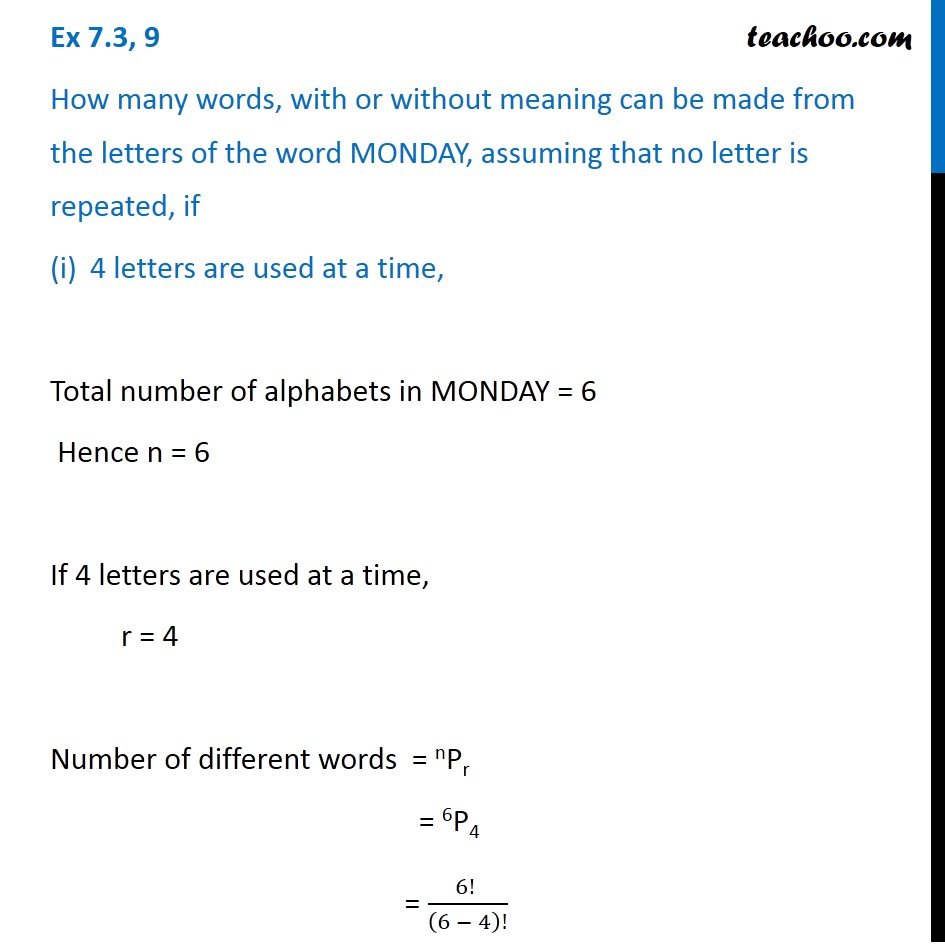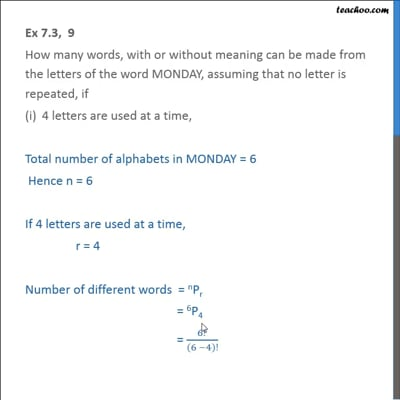This video is only available for Teachoo black users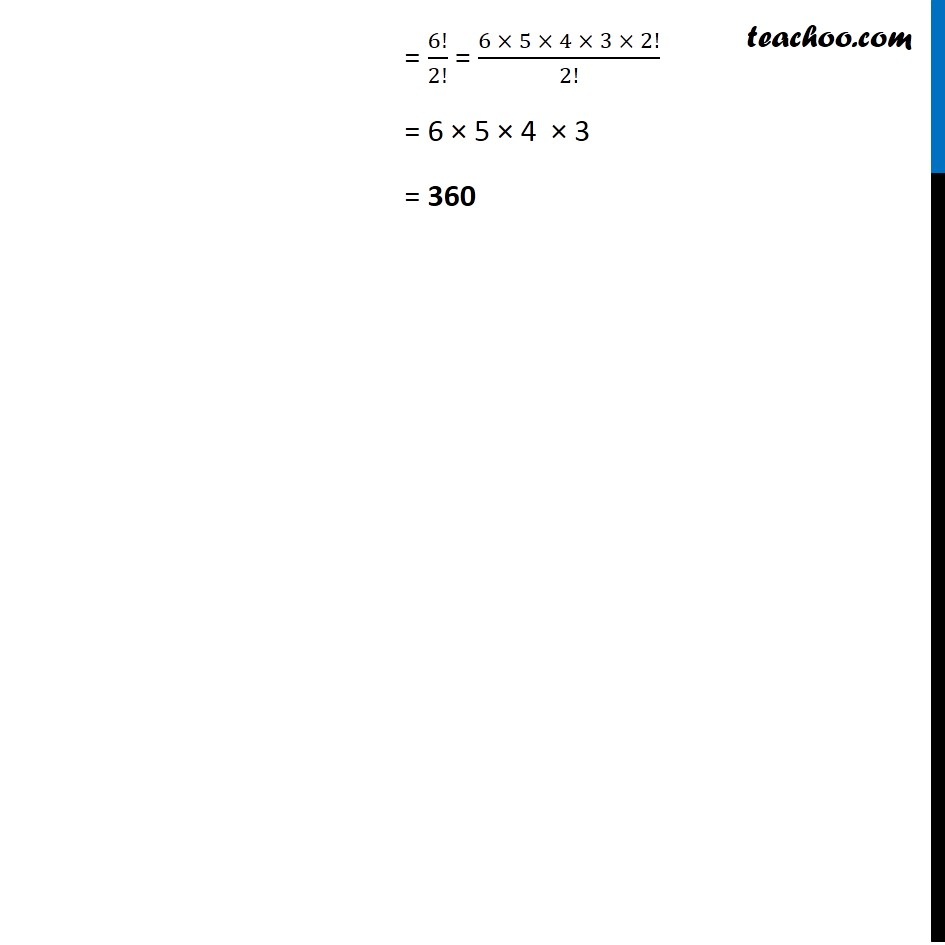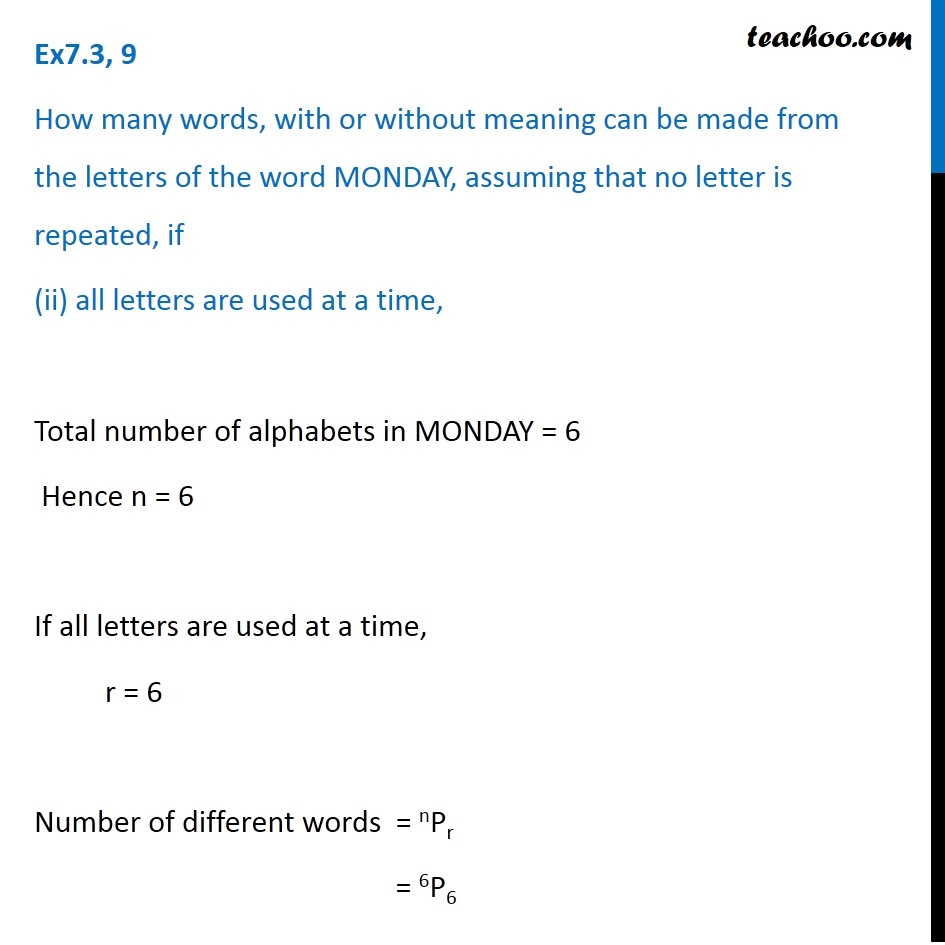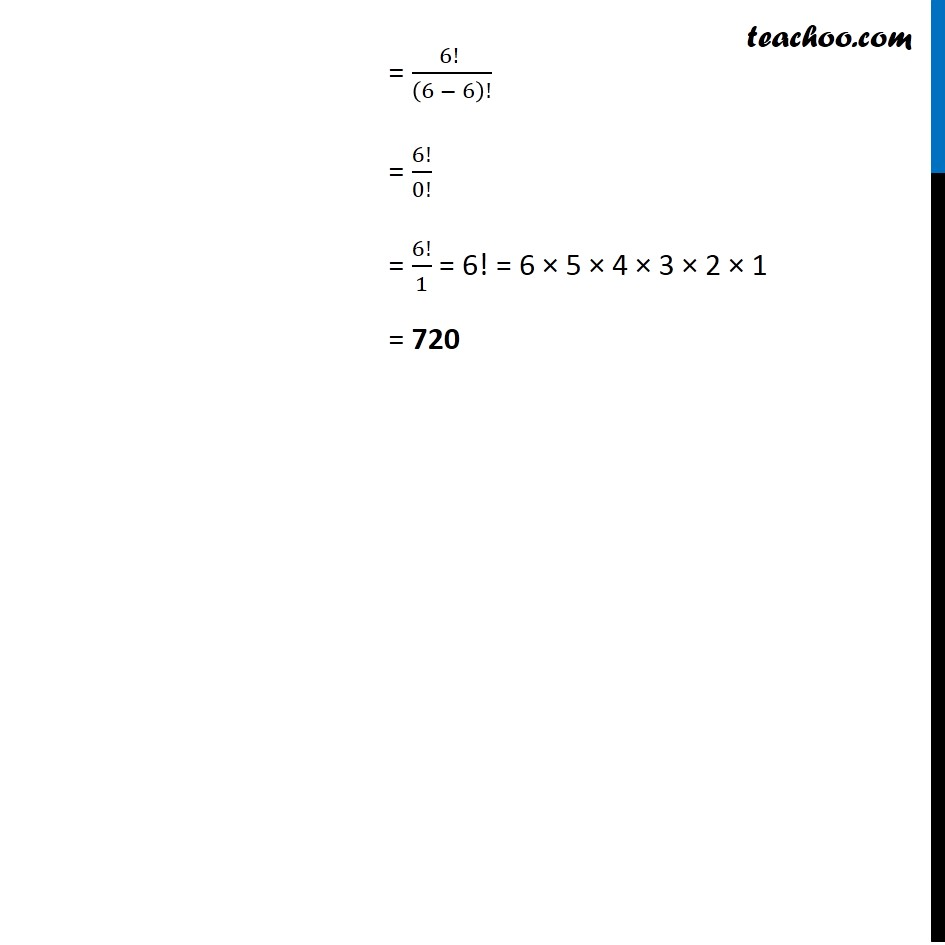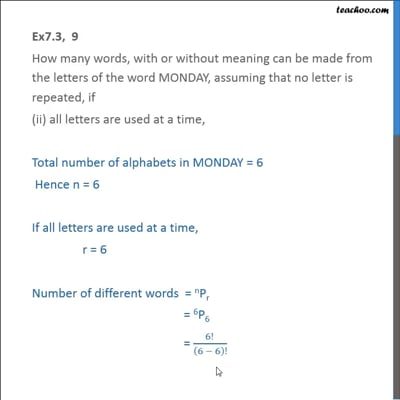This video is only available for Teachoo black users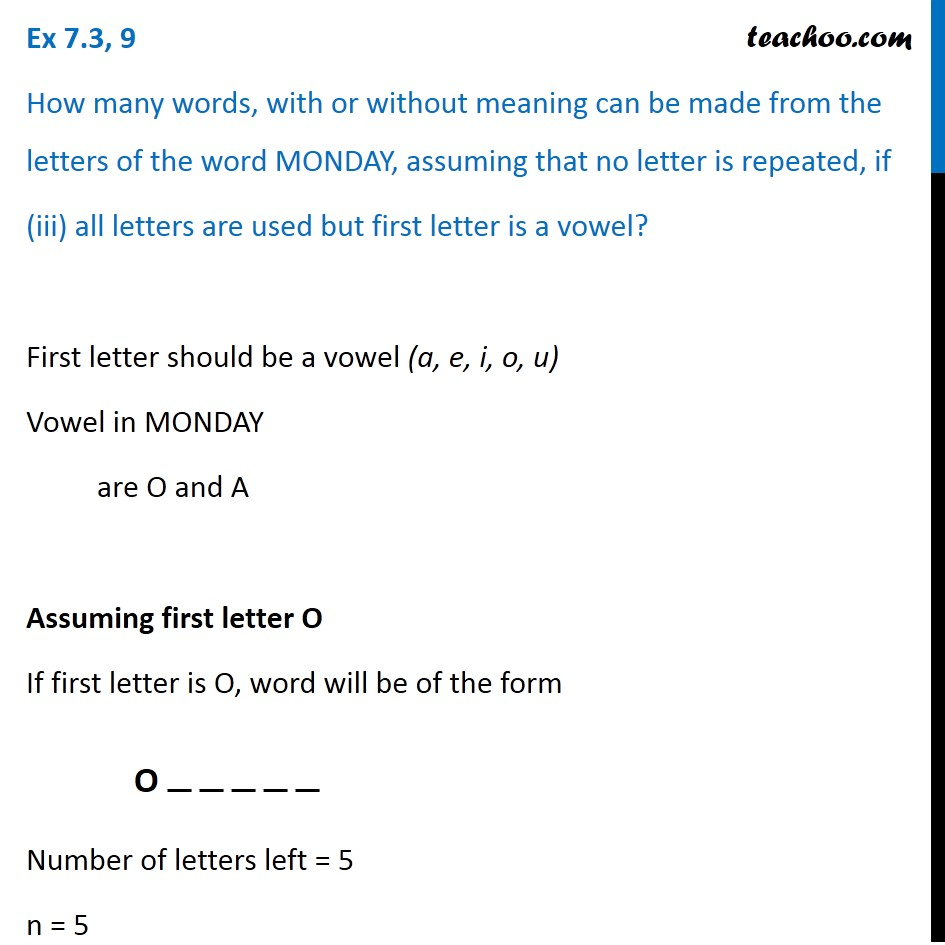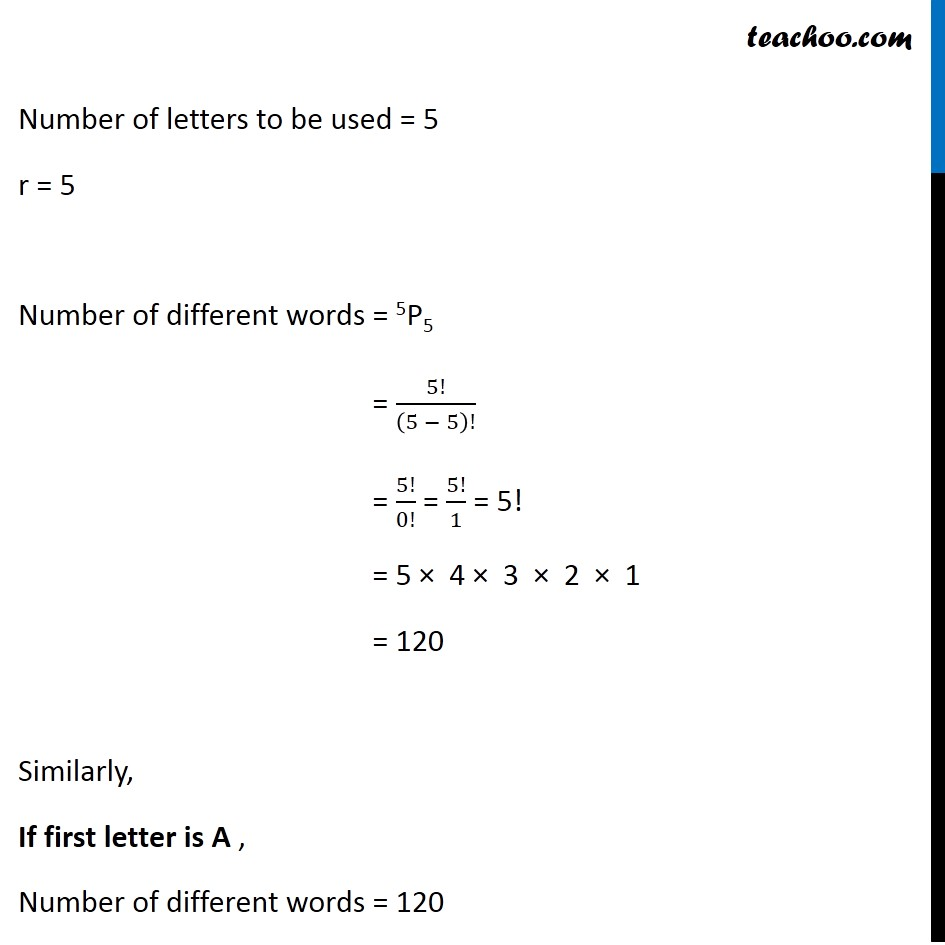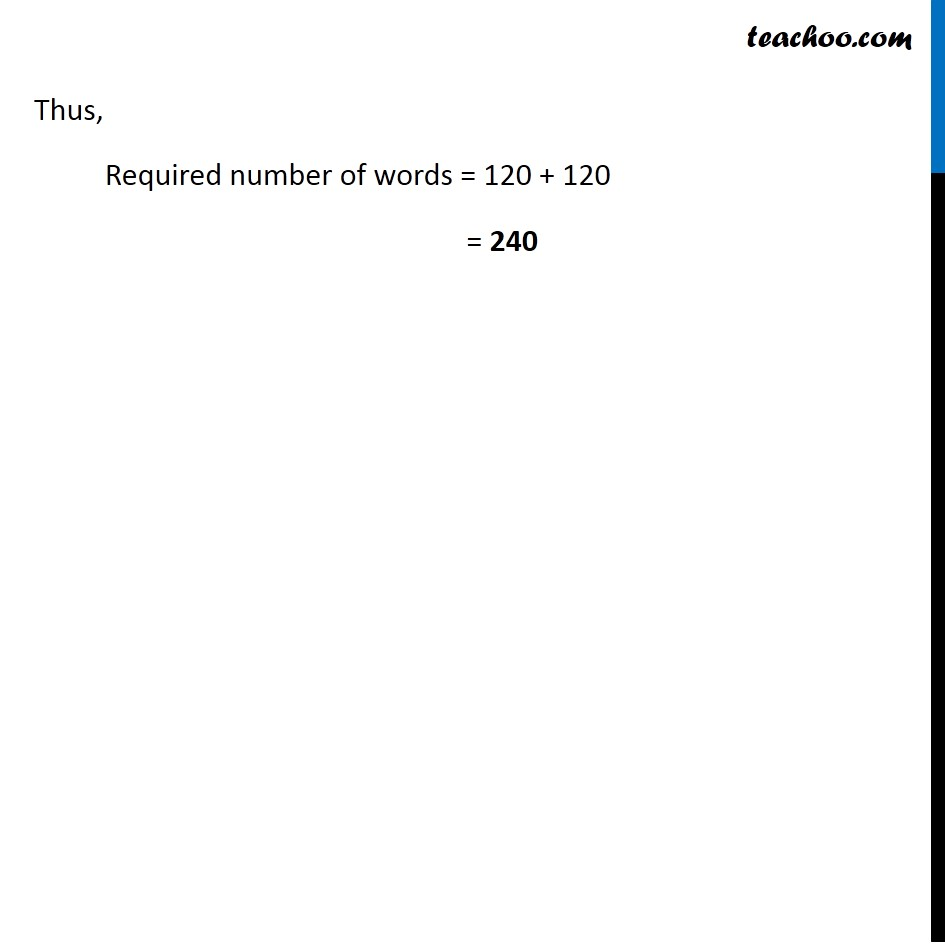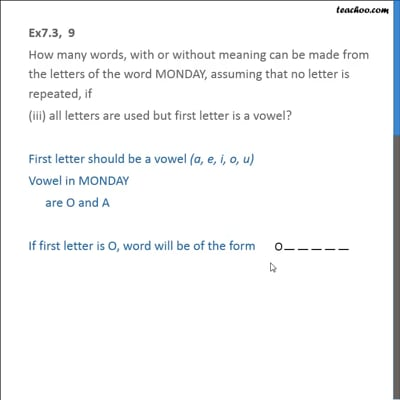This video is only available for Teachoo black users

Solve all your doubts with Teachoo Black (new monthly pack available now!)

### Transcript

Ex 7.3, 9 How many words, with or without meaning can be made from the letters of the word MONDAY, assuming that no letter is repeated, if 4 letters are used at a time, Total number of alphabets in MONDAY = 6 Hence n = 6 If 4 letters are used at a time, r = 4 Number of different words = nPr = 6P4 = (6! )/(6 − 4)! = 6!/2! = (6 × 5 × 4 × 3 × 2!)/2! = 6 × 5 × 4 × 3 = 360 Ex7.3, 9 How many words, with or without meaning can be made from the letters of the word MONDAY, assuming that no letter is repeated, if (ii) all letters are used at a time, Total number of alphabets in MONDAY = 6 Hence n = 6 If all letters are used at a time, r = 6 Number of different words = nPr = 6P6 = (6! )/(6 − 6)! = 6!/0! = 6!/1 = 6! = 6 × 5 × 4 × 3 × 2 × 1 = 720 Ex 7.3, 9 How many words, with or without meaning can be made from the letters of the word MONDAY, assuming that no letter is repeated, if (iii) all letters are used but first letter is a vowel? First letter should be a vowel (a, e, i, o, u) Vowel in MONDAY are O and A Assuming first letter O If first letter is O, word will be of the form Number of letters left = 5 n = 5 Number of letters to be used = 5 r = 5 Number of different words = 5P5 = 5!/(5 − 5)! = 5!/0! = 5!/1 = 5! = 5 × 4 × 3 × 2 × 1 = 120 Similarly, If first letter is A , Number of different words = 120 Thus, Required number of words = 120 + 120 = 240